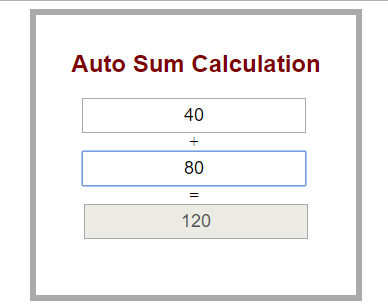## AUTO SUM CALCULATION USING JAVASCRIPT

This JQuery script will show how to auto calculate sum while you type values in the fields. It’s a Simple Program for beginners. There’s nothing much in this program, user types a certain value in empty TextBox after that, the sum will automatically displ...

Type : Project

File Size : 32.7 KB# Similar Projects and Reports

#### AUTO SUM CALCULATION USING JAVASCRIPT

This JQuery script will show how to auto calculate sum while you type values in the fields. It’s a Simple Program for beginners. There’s nothing much in this program, user types a certain value in empty TextBox after that, the sum will automatically displ...

#### CALCULATOR USING JAVASCRIPT

All our calculator will do is basic arithmetic: add, subtract, multiply and divide. It includes a display area of numbers, numeric buttons 0-9, decimal point button, function buttons(+,-,*,/ & C), and Calculate button ( = ).

#### Calculator In HTML Using JavaScript With Source Code

This Calculator is a simple project in HTML5, CSS, and JavaScript. This project is for solving the mathematical calculations of numbers. You can see the basic features of the calculator in this project.

#### JavaScript Calculator With Source Code

This javascript calculator project is a simple project in HTML5, CSS, and JavaScript. Here, the calculator functions as that of a real-life simple calculator. This project is for solving the mathematical calculations of numbers. You can see the basic feat...

#### NUMBER CONVERSIONS USING JAVASCRIPT

This program coverts Decimal number to binary, hexadecimal and octal.

#### HOTEL SITE USING HTML, JAVASCRIPT & CSS

This Project is developed using HTML, JAVASCRIPT & CSS. Here, customers can check the facilities provided by the Hotel. There is Gallery section to check the Images of particular places of the Hotel. Staff’s Detail information are also provided on Website...